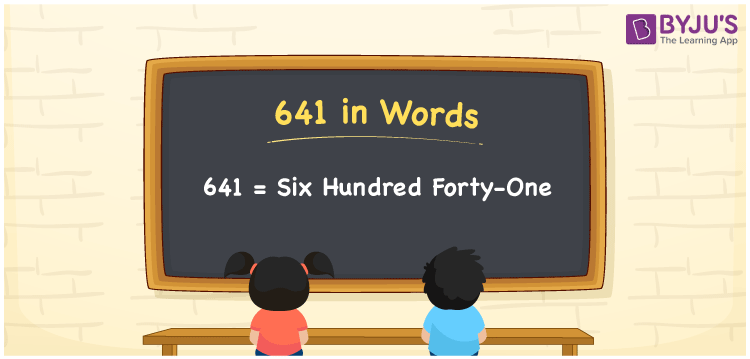# 641 in Words

We can write 641 in words as Six hundred forty-one. If you marked Rs. 641, on an invoice, then you may read it as, “Six hundred forty-one rupees”. Thus, to spell the given cardinal number or communicate the given numerical amount, we generally use the word form of that particular number. Here, Six hundred forty-one is the word form of 641 and is also called the number name.

 641 in words Six hundred forty-one Six hundred forty-one in Numbers 641

## 641 in English Words

We generally express numbers in words using the English alphabet. So, we spell 641 in English words as “Six hundred forty-one”.## How to Write 641 in Words?

Let us create a place value chart with three columns to convert the number 641 into words, as shown below.

 Hundreds Tens Ones 6 4 1

Here, ones = 1, tens = 4, hundreds = 6

By expanding these digits, we get;

6 × Hundred + 4 × Ten + 1 × One

= 6 × 100 + 4 × 10 + 1 × 1

= 600 + 40 + 1

= 600 + 41

= Six hundred + Forty-one

= Six hundred forty-one

Therefore, 641 in words = Six hundred forty-one

As we know, 641 is a natural number that precedes 642 and succeeds 640.

641 in words – Six hundred forty-one

Is 641 an even number? – No

Is 641 an odd number? – Yes

Is 641 a perfect square number? – No

Is 641 a perfect cube number? – No

Is 641 a prime number? – Yes

Is 641 a composite number? – No

## Frequently Asked Questions on 641 in Words

Q1

### How do you write 641 in word form?

We can write the number 641 in word form as Six hundred forty-one.
Q2

### Express 641.25 in words.

641.25 can be written in words as “Six hundred forty-one point two five”.
Q3

### Write the value of 234 + 641 in words.

234 + 641 = 875 Thus, the value of 234 + 641, i.e., 875 in words is Eight hundred seventy-five.